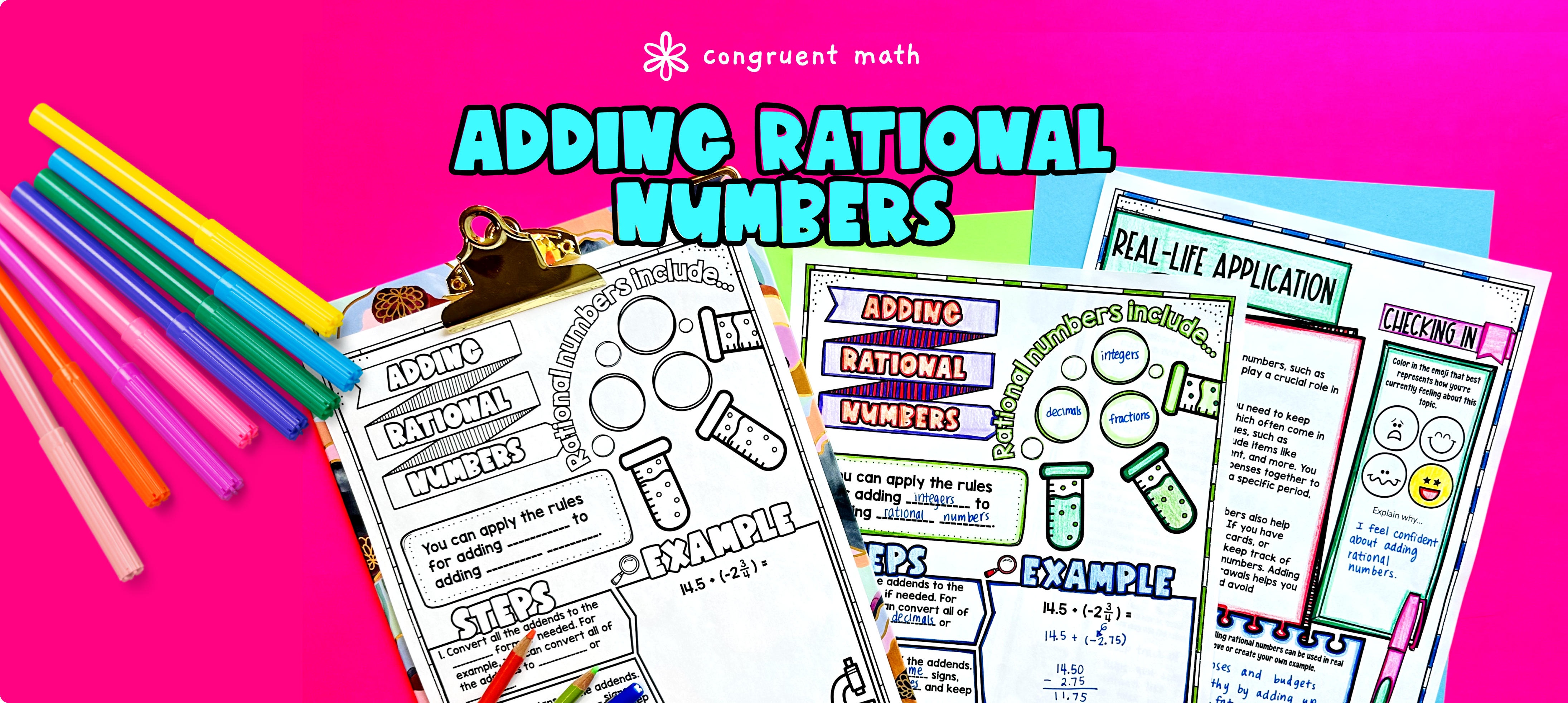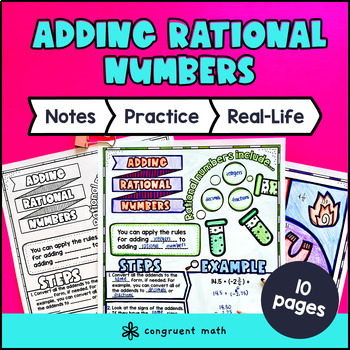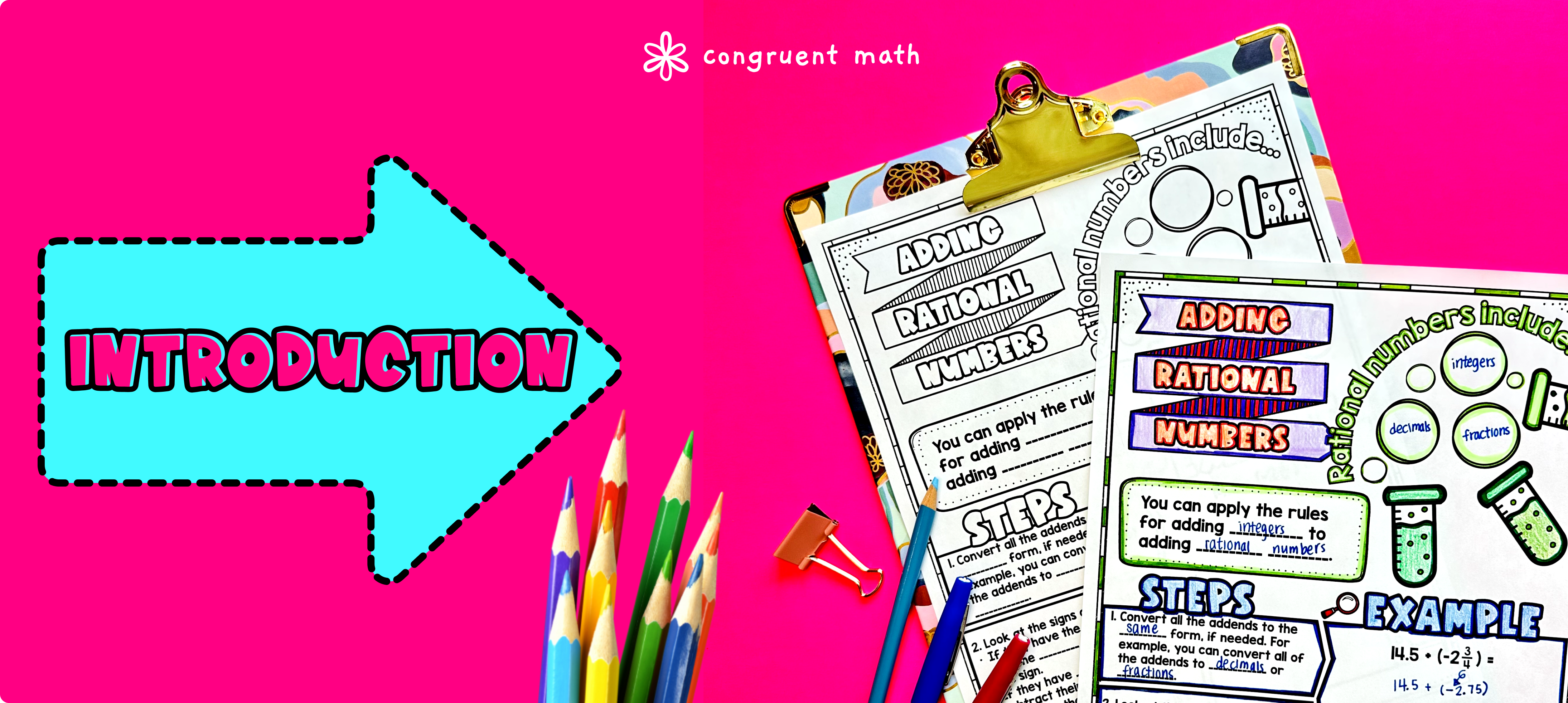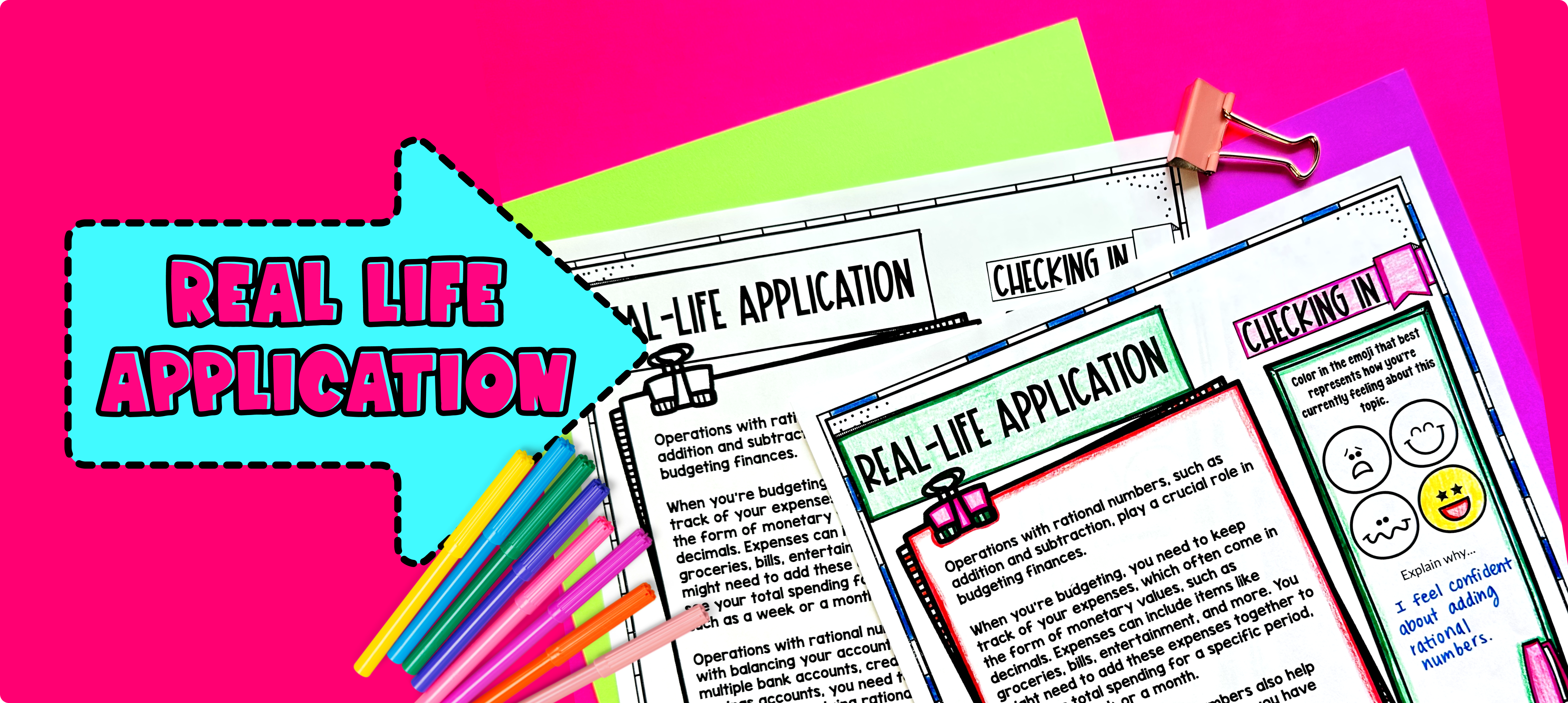# Adding Rational Numbers Lesson PlanPin This

## Overview

In this lesson plan, students will learn about adding rational numbers (negative fractions, decimals, integers) and their real-life applications. Through artistic, interactive guided notes, check for understanding, practice sheets, and a color by number activity, students will gain a comprehensive understanding of adding rational numbers.

The lesson culminates with a real-life example that explores how adding rational numbers can be used for budgeting travel expenses and balancing accounts.

## Get the Lesson Materials\$4.25

## Learning Objectives

After this lesson, students will be able to:

• Add rational numbers, including negative fractions, decimals, and integers

• Convert between rational numbers formats (optional)

• Understand the real-life applications of adding rational numbers, such as budgeting for travel expenses and balancing accounts

## Prerequisites

Before this lesson, students should be familiar with:

• Basic addition and subtraction skills for positive fractions, decimals, and integers

• Understanding of mixed numbers (mixed fractions)

## Key Vocabulary

• Rational Numbers

• Negative Fractions

• Negative Decimals

• Negative Integers

• Absolute Values

## Procedure

### IntroductionPin This

As a hook, ask students why adding rational numbers is important in everyday life. For example, how might adding negative integers be useful when keeping track of your spending? In this lesson, we will explore the concept of adding rational numbers, including negative fractions, decimals, and integers. We will also see how adding rational numbers can be used in real-life situations, such as budgeting for travel expenses and balancing accounts.

Follow the guided notes to introduce the following: What are rational numbers, how are the rules for rational numbers related to the properties of integers, signs for rational number addition, steps to add rational numbers, and key example (pg. 1 of guided notes).

Walk through the key points of each topic and refer to the FAQ below for a walkthrough on this, as well as ideas on how to respond to common student questions. On the following page, students will have the opportunity to practice with different types of rational numbers, including fractions, integers, and decimals (pg. 2 of guided notes). Call on students to talk through their answers, potentially on the whiteboard or projector. Based on student responses, reteach concepts that students need extra help with.

### PracticePin This

Have students practice adding rational numbers using the Integers Decimals Fractions Sheet. Walk around to answer student questions.

Fast finishers can dive into the practice sheet with problems instead of individual bubbles (pg. 3 of guided notes) or color by number (pg. 4 of guided notes) for extra practice. You can assign it as homework for the remainder of the class.

### Real-Life ApplicationPin This

Bring the class back together, and introduce the concept of budgeting for travel expenses. Explain how adding rational numbers can be used to calculate expenses and keep track of spending. Ask students to brainstorm other real-life situations where adding rational numbers might be useful, such as balancing a checkbook or calculating the total cost of items in a shopping cart. Refer to the FAQ for more ideas on how to teach it!” Stop after writing this section and do not generate the rest of the document.

## Extensions

If you're looking for digital practice for adding and subtracting rational numbers, try these Pixel Art activities in Google Sheets. Every answer is automatically checked, and correct answers unlock parts of a mystery picture. It's incredibly fun, and a powerful tool for differentiation.

Here are 2 activities to explore: